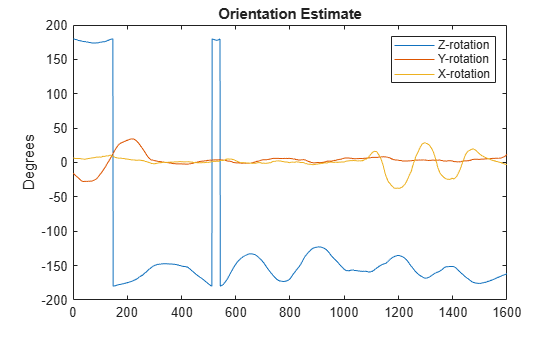Main Content

# complementaryFilter

Orientation estimation from a complementary filter

## Description

The `complementaryFilter` System object™ fuses accelerometer, gyroscope, and magnetometer sensor data to estimate device orientation and angular velocity.

To estimate orientation using this object:

1. Create the `complementaryFilter` object and set its properties.

2. Call the object with arguments, as if it were a function.

To learn more about how System objects work, see What Are System Objects?.

## Creation

### Syntax

``FUSE = complementaryFilter``
``FUSE = complementaryFilter('ReferenceFrame',RF)``
``FUSE = complementaryFilter(___,Name,Value)``

### Description

````FUSE = complementaryFilter` returns a `complementaryFilter` System object, `FUSE`, for sensor fusion of accelerometer, gyroscope, and magnetometer data to estimate device orientation and angular velocity. ```
````FUSE = complementaryFilter('ReferenceFrame',RF)` returns a `complementaryFilter` System object that fuses accelerometer, gyroscope, and magnetometer data to estimate device orientation relative to the reference frame `RF`. Specify `RF` as `'NED'` (North-East-Down) or `'ENU'` (East-North-Up). The default value is `'NED'`.```

example

````FUSE = complementaryFilter(___,Name,Value)` sets each property `Name` to the specified `Value`. Unspecified properties have default values.```

## Properties

expand all

Unless otherwise indicated, properties are nontunable, which means you cannot change their values after calling the object. Objects lock when you call them, and the `release` function unlocks them.

If a property is tunable, you can change its value at any time.

For more information on changing property values, see System Design in MATLAB Using System Objects.

Input sample rate of the sensor data in Hz, specified as a positive scalar.

Tunable: No

Data Types: `single` | `double`

Accelerometer gain, specified as a real scalar in the range of [0, 1]. The gain determines how much the accelerometer measurement is trusted over the gyroscope measurement for orientation estimation. This property is tunable.

Data Types: `single` | `double`

Magnetometer gain, specified as a real scalar in the range of [0, 1]. The gain determines how much the magnetometer measurement is trusted over the gyroscope measurement for orientation estimation. This property is tunable.

Data Types: `single` | `double`

Enable magnetometer input, specified as `true` or `false`.

Data Types: `logical`

Output orientation format, specified as `'quaternion'` or `'Rotation matrix'`. The size of the output depends on the output orientation format:

• `'quaternion'` –– Output is an N-by-1 `quaternion`.

• `'Rotation matrix'` –– Output is a 3-by-3-by-N rotation matrix.

N is the number of samples.

Data Types: `char` | `string`

## Usage

### Syntax

``[orientation,angularVelocity] = FUSE(accelReadings,gyroReadings,magReadings)``
``[orientation,angularVelocity] = FUSE(accelReadings,gyroReadings)``

### Description

example

````[orientation,angularVelocity] = FUSE(accelReadings,gyroReadings,magReadings)` fuses accelerometer, gyroscope, and magnetometer data to compute orientation and angular velocity. To use this syntax, set the `HasMagnetometer` property as `true`.```
````[orientation,angularVelocity] = FUSE(accelReadings,gyroReadings)` fuses accelerometer and gyroscope data to compute orientation and angular velocity. To use this syntax, set the `HasMagnetometer` property as `false`.```

### Input Arguments

expand all

Accelerometer readings in the sensor body coordinate system in m/s2, specified as an N-by-3 matrix. N is the number of samples, and the three columns of `accelReadings` represent the [x y z] measurements. Accelerometer readings are assumed to correspond to the sample rate specified by the `SampleRate` property. In the filter, the gravity constant g is assumed to be `9.81` m/s2.

Data Types: `single` | `double`

Gyroscope readings in the sensor body coordinate system in rad/s, specified as an N-by-3 matrix. N is the number of samples, and the three columns of `gyroReadings` represent the [x y z] measurements. Gyroscope readings are assumed to correspond to the sample rate specified by the `SampleRate` property.

Data Types: `single` | `double`

Magnetometer readings in the sensor body coordinate system in µT, specified as an N-by-3 matrix. N is the number of samples, and the three columns of `magReadings` represent the [x y z] measurements. Magnetometer readings are assumed to correspond to the sample rate specified by the `SampleRate` property.

Data Types: `single` | `double`

### Output Arguments

expand all

Orientation that rotates quantities from the local navigation coordinate system to the body coordinate system, returned as quaternions or an array. The size and type of `orientation` depends on whether the `OrienationFormat` property is set to `'quaternion'` or `'Rotation matrix'`:

• `'quaternion'` –– the output is an N-by-1 vector of quaternions, where N is the number of samples.

• `'Rotation matrix'` –– the output is a 3-by-3-by-N array of rotation matrices, where N is the number of samples.

Data Types: `quaternion` | `single` | `double`

Angular velocity expressed in the sensor body coordinate system in rad/s, returned as an N-by-3 array, where N is the number of samples.

Data Types: `single` | `double`

## Object Functions

To use an object function, specify the System object as the first input argument. For example, to release system resources of a System object named `obj`, use this syntax:

`release(obj)`

expand all

 `step` Run System object algorithm `release` Release resources and allow changes to System object property values and input characteristics `reset` Reset internal states of System object `clone` Create duplicate System object `isLocked` Determine if System object is in use

## Examples

collapse all

Load the `rpy_9axis` file, which contains recorded accelerometer, gyroscope, and magnetometer sensor data from a device oscillating in pitch (around y-axis), then yaw (around z-axis), and then roll (around x-axis). The file also contains the sample rate of the recording.

```ld = load('rpy_9axis.mat'); accel = ld.sensorData.Acceleration; gyro = ld.sensorData.AngularVelocity; mag = ld.sensorData.MagneticField;```

Create a complementary filter object with sample rate equal to the frequency of the data.

```Fs = ld.Fs; % Hz fuse = complementaryFilter('SampleRate', Fs);```

Fuse accelerometer, gyroscope, and magnetometer data using the filter.

`q = fuse(accel, gyro, mag);`

Visualize the results.

```plot(eulerd( q, 'ZYX', 'frame')); title('Orientation Estimate'); legend('Z-rotation', 'Y-rotation', 'X-rotation'); ylabel('Degrees');```Valenti, R., I. Dryanovski, and J. Xiao. "Keeping a good attitude: A quaternion-based orientation filter for IMUs and MARGs." Sensors. Vol. 15, Number 8, 2015, pp. 19302-19330.

## Support

#### Sensor Fusion and Tracking for Autonomous Systems

Download white paper